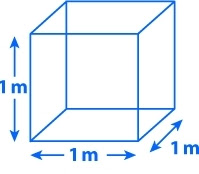# Easy Method of CBM Calculation Under LCL Sea Shipment

By | July 4, 2015

### Easy Method of CBM Calculation Under LCL Sea Shipment

Mayedul Islam
Merchandiser at Fashion Xpress Buying House.
Email: mayedul.islam66@gmail.com

#### Introduction:

A freight forwarder charges the freight cost on the basis of CBM. CBM means cubic meter. In garments sector, it’s a known term for all the merchandisers. Accurate CBM calculation has a great importance on reducing shipping cost. Because, if the cargo is full container loaded (FCL), then you have to pay the freight charge in full container load (FCL) basis, but if the cargo is less container loaded then you have to pay the freight charge in Less container load (LCL) basis. As its importance in merchandising, today I will discuss about the all parts of CBM (Cubic Meter) calculation.CBM (Cubic Meter)

#### CBM Calculation Under LCL Sea Shipment:

Before starting the CBM calculation, at first you have to find out the length, width and height of a carton, here you should also find out the total quantity of carton for an order.

If the length, width and height measurements are in “inch” unit, then you have to convert these into “centimeter (cm)” unit by multiplying 2.54 (as we know, 1 inch=2.54cm).

After converting from “inch” unit to “centimeter (cm)” unit, now you have to convert these into “meter (m)” unit by dividing 100 (as we know, 1m=100cm).

Now, yours all length, width and height are in “meter (m)” unit.

In this situation, you must follow the following formula,

CBM calculation for 1 carton = Length (m) × width (m) × height (m)…………… (1)

CBM calculation for total carton of an order = CBM calculation for 1 carton × total number of carton quantity ……………… (2)

Now I will clear all the above explanation by providing an example with solution.

#### Example of CBM Calculation:

Suppose,

Total cartoon quantity of an order is 5000 (Every carton is in sane measurement).

• Length for each carton is 22”,
• Width for each carton is 15”,
• Height for each carton is 6”.

Now, calculate the CBM for shipping an order.

Solution:

Carton length = 22” = (22 × 2.54) cm = 55.88cm = (55.88/100) m = 0.5588m.

Carton width = 15” = (15 × 2.54) cm = 38.1cm = (38.1/100) m = 0.381m.

Carton height = 6” = (6 × 2.54) cm = 15.24 cm = (15.24/100) m =0.1524m.

Now by applying formula number (1), we have-

CBM calculation for 1 carton = Length (m) × width (m) × height (m)
= (0.5588 × 0.381 × 0.1524) m3
= 0.03244639 m3

Now finally applying formula number (2), we have-

CBM calculation for total cartoon = CBM calculation for 1 carton × total number of carton quantity
= (0.03244639 × 5000) m3
=162.23195 m3

So, CBM calculation for 5000 carton is 162.23195 m.

## 7 thoughts on “Easy Method of CBM Calculation Under LCL Sea Shipment”

1.Anurag Singh

Can you please tell me that how we reduce the Alteration in garment production.

1.Mayedul

keep in touch……….
U will get it very soon……..

2.Kamrul

To me this method is critical. You can try this as follows. Say carton measurement is L-40xW-25xH-15. See below pls.

.40x.25x.14xcarton qty= RESULT.

1.Mayedul

3.Gaby

I actually watend to construct a brief remark so as to appreciate you for some of the nice tips you are writing here. My time consuming internet lookup has now been compensated with brilliant facts and strategies to write about with my family. I d repeat that we website visitors are undeniably blessed to live in a wonderful website with so many perfect people with great hints. I feel rather fortunate to have discovered your entire weblog and look forward to so many more cool minutes reading here. Thank you once again for a lot of things.

1.Mayedul Islam

4.Md. Masum Billah